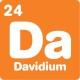# What's new## The triple bounce

, 1 May 2019

I’m thinking of a two digit number.

If you add the digits together and then multiply by three, you get the number back again!

What number am I thinking of?There are a lot of ways to tackle this question. You could just start checking two digit numbers, but that could take a while.

There are a few smart observations you can make to simplify your search. Here are a few we found:

• Adding together each of the digits in a number gives that number’s digit sum. The biggest digit sum you can make with two digits is 18: that’s the digit sum of 99, as 9 + 9 = 18. The number I’m thinking of can’t be any bigger than three times that, which is 54.
• The number has to be divisible by three, because it’s equal to the digit sum times three.
• There is a rule that any number that is divisible by three, has a digit sum that is also divisible by three. In this case, the number we’re looking for is the digit sum (which is divisible by three), times three. So it must be divisible by nine. There are only a few two digit numbers that are under 54 and divisible by 9.

A bit of searching will find the only answer: 27.

For more brainteasers and puzzles for kids, subscribe to Double Helix magazine!Categories:

## Similar posts

1.Why ignore algebra? 3(a+b)=10a+b It is important that place value is recognised and can be represented in algebra. 2b=7a so b/7=a/2 and for digits a and b to be whole numbers, the result follows.

Thank you for these brainteasers!

2.Hi Paul,
Nice answer! One reason I steer clear of algebra is I know someone will always do it for me in the comments 😉

If you want more things to play with, try changing the multiple (x3) in this puzzle. There’s an interesting pattern to uncover!

3.Within the problem parameters number “00” works also. Love all your emails puzzles, quizzes and comments etc. But you send them out at a crazy time, a little east coast USA humor. I hope to visit soon.

4.Yes, there are unique solutions (all are multiples of 9) for multiplication by 2, 3, 5, 6, 8 and 9.

Curiously, all cubes are also roughly (+/- 1) multiples of 9. (Discussed in my book on Proof: Interesting activities in conjecture and mathematical proof http://www.pbperth.com )

Paul